# Radio Waves, Radiation and Reception of

## Radio Waves, Radiation and Reception of

The radiation of radio waves is the process of excitation of traveling electromagnetic waves in the radio band in the space surrounding the source of oscillations of a current or charge. In the process the energy of the source is transformed into the energy of the electromagnetic waves that are propagating in space. The process of reception of radio waves is the inverse of their radiation; it consists in the conversion of the energy of electromagnetic waves into the energy of an alternating current. Radio waves are radiated and received by means of transmitting and receiving antennas.

Radiation. Alternating currents traveling through conductors, as well as alternating fields, may be the source of primary electric oscillations. However, alternating currents of relatively low frequency, such as the industrial frequency of 50 hertz (Hz), are unsuitable for radiation: at such frequencies an efficient radiator cannot be devised. Indeed, if the electric oscillations occur, for example, in an inductance coil whose dimensions are small in comparison with the wavelength λ that corresponds to the frequency of oscillation of the current flowing in the coil, then in the coil turn, for each section with a current flowing in one direction, such as A (Figure 1), there exists another section B, at a distance less than λ/2 from A, in which the direction of the current at the same instant is opposite. At great distances from the turn the waves radiated by elements A and B attenuate each other. Since the turn consists of such pairs of antiphase elements, it—and consequently the entire coil—radiates poorly. An oscillatory circuit containing an inductance coil and a capacitor also radiates poorly. At each instant the charges on the capacitor plates are of equal magnitude and opposite sign and separated from each other by a distance much less than λ/2.Figure 1. Winding of an inductance coil

From this it follows that an open circuit, in which either there are no sections with antiphase oscillations of current or charge or the distance between them is not small compared to λ/2, is necessary for efficient radio wave radiation. If the dimensions of the circuit are such that the time required for propagation of changes in the electromagnetic field in the circuit is comparable to the period of oscillations of the current or charge (the velocity of propagation of perturbations is finite), then the conditions for a quasi-stationary process are not met, and part of the energy of the source departs in the form of electromagnetic waves. For practical purposes electromagnetic waves with λ < 10 km are usually used.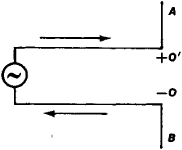Figure 2. Electric dipole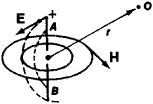Figure 3. Structure of electric field E and magnetic field H near a dipole: the broken lines indicate the lines of force of the electric field, and the fine solid lines indicate the lines of force of the magnetic field; O is the point of observation.

Radiators. The simplest radio-wave radiator consists of two segments A and B of a rectilinear conductor attached to the ends OO’ of a double line along which the electromagnetic wave is propagating (Figure 2). Motion of charges, or an alternating current, arises in segments A and B under the action of the electric field of the wave. At each moment the charges at points O and O’ are of equal magnitude and opposite sign—that is, segments A and B form an electric dipole, which determines the configuration of the electric field it generates. On the other hand, the currents in segments A and B coincide in direction, and therefore the lines of force of the magnetic field, as in the case of a rectilinear current, are circular (Figure 3). Thus, an electromagnetic field in which the fields E and H are perpendicular to each Other arises in the space surrounding the dipole. The electromagnetic field propagates in space, moving away from the dipole (Figure 4).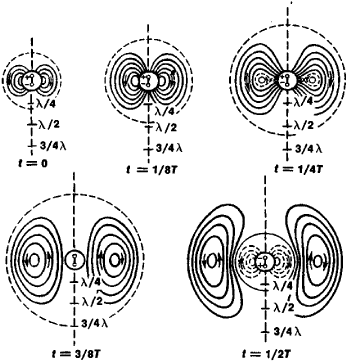Figure 4. Instantaneous patterns of electric lines of force near a dipole for time intervals separated by one-eighth of the period T of the current oscillations

The waves radiated by the dipole have a definite polarization. The intensity vector of the electric field E of the wave at the point of observation O (Figure 3) lies in a plane that passes through the dipole and the radius vector r, which is drawn from the center of the dipole to the point of observation. The magnetic field vector H is perpendicular to the plane.

An alternating electromagnetic field arises throughout the entire space surrounding the dipole and propagates in all directions from the dipole. The dipole radiates a spherical wave, which at a great distance from the dipole may be considered plane (locally plane). However, the intensity of the electric and magnetic fields generated by the dipole, and consequently the radiated energy, is different in different directions. It is greatest in directions perpendicular to the dipole and gradually decreases to zero along the axis of the dipole. The dipole virtually does not radiate in this direction. The distribution of the radiated power in different directions is characterized by the radiation pattern. A three-dimensional dipole radiation pattern is toroidal in shape.

The total power radiated by a dipole depends on the power supplied and the ratio between the dipole’s length l and the wavelength λ. For a dipole to radiate a significant portion of the power fed to it, its length should not be small compared to λ/2. The difficulty of radiating very long waves is associated with this fact. If / is properly selected and the energy losses to heating the conductors of the dipole and line are small, most of the power from the source is expended on radiation. Thus, a dipole is a consumer of the power from the source, similar to an active resistance that is connected to the end of the line and consumes the power supplied. In this sense a dipole has a radiation resistance Rrad, which is equal to the active resistance in which the same power would be consumed.

The dipole described above is the simplest transmitting antenna and is called a center-face dipole. Such a dipole was first used by H. Hertz (1888) in experiments that detected the existence of radio waves. In a Hertz dipole the electrical oscillations were excited by means of spark discharge—the only source of electric oscillations known at the time. In addition to the center-face dipole, the asymmetric dipole, which is excited at its end and radiates uniformly in a horizontal plane, is also used (for longer waves).

In addition to wire antennas (wire dipoles), other types of radio-wave radiators exist. The magnetic antenna has come into wide use. It is a rod made of magnetic material with high magnetic permeability μ, onto which is wound a coil consisting of fine wire. According to the principle of duality, the lines of force of the magnetic field of a magnetic antenna will duplicate the pattern of the lines of force of the electric field of a wire dipole (Figure 5. a and b).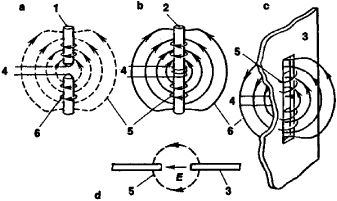Figure 5. Comparison of an electric dipole (a) and a magnetic radiator (b) and slot radiators (c) and (d): (1) current-carrying wire, (2) core made of a material with high magnetic permeability, (3) metal screen in which a slot is cut, (4) wires from high-frequency electric generator, (5) lines of force of electric field, (6) lines of force of magnetic field

If a slit is cut in the walls of a radio wave guide or cavity resonator in which alternating superhigh-frequency surface currents are flowing, in such a way that the slit intersects the direction of the current, the distribution of the currents is distorted sharply, the shielding is disrupted, and the electromagnetic energy is radiated outward. The distribution of the fields of a slot radiator is similar to the distribution of the fields of a magnetic antenna. Therefore a slot radiator is called a magnetic dipole (Figure 5, c and d). The radiation pattern of magnetic and slot radiators, like the pattern of an electric dipole, is a toroid.

More directional radiation is generated by antennas that consist of several wire or slot radiators. This is a result of interference of the radio waves radiated by the individual radiators. If the currents feeding them have identical amplitude and phase (uniform in-phase excitation), at a sufficiently great distance in a direction perpendicular to the radiating surface the waves from the individual radiators have identical phases and produce maximum radiation. The field generated in other directions is much weaker. Some increase in field intensity takes place in directions for which the difference between the phases of the waves arriving from the outermost radiators is equal to (n + 1) π/2, where n is a whole number. In this case a planar cross section of the radiation pattern contains several lobes, the largest of which is called the major lobe and corresponds to the maximum radiation; the others are called side lobes.

In modern technology antenna arrays containing as many as 1,000 radiators are used. The surface on which they are located is called the antenna aperture and may have any form. By assigning different distribution of current amplitudes and phases at the aperture it is possible to obtain a radiation pattern of any shape. The in-phase excitation of the radiators that form the flat grid makes possible the production of very high directivity of radiation, and a change in the distribution of the current at the aperture creates the possibility of changing the shape of the radiation pattern.

To increase the directivity of radiation, which is characterized by the width of the main lobe, the size of the antenna must be increased. The relation between the width of the major lobe 6, the largest dimension of the aperture L, and the radiated wave-lenght λ is defined by the formulas

θ=Cλ/2

for in-phase excitation and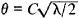if the radiators lie along a certain axis and the phase shift within them is chosen such that the maximum radiation is directed along this axis. In the above formulas, C represents constants that depend on the distribution of current amplitude over the aperture.

If radio wave guide gradually expands to an open end in the form of a funnel or horn, a wave in the wave guide is gradually transformed into a wave characteristic of free space. Such a horn antenna produces directional radiation.

Very high radiation directivity (as narrow as hundredths of a degree for decimeter and shorter waves) can be achieved by means of reflector and lens antennas. In such antennas, the spherical wave front radiated by an electric or magnetic dipole or by a horn radiator is transformed into a plane front as a result of reflection and refraction. However, because of wave diffraction, the pattern in this case also has major and minor directivity lobes. A reflector antenna is a metallic mirror (1), most often in the form of a paraboloid of revolution or a parabolic cylinder at whose focus the primary radiator is located (Figure 6). The lenses for the radio waves are three-dimensional grids consisting of metal balls, rods, and other items (artificial dielectrics) or a set of rectangular wave guides.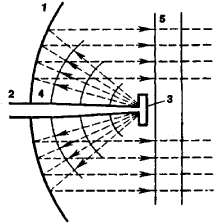Figure 6. Diagram of a reflector antenna: (1) parabolic reflector, (2) wave guide connecting two-slot radiator (3) with the generator, (4) spherical wave front formed by the radiator, (5) flat wave front after reflection from the mirror

Reception. Any transmitting antenna may serve as a receiving antenna. If a wave propagating in space acts on an electric dipole, its electric field excites in the dipole current oscillations, which are then amplified and transformed in frequency and act on the output devices. Dipole radiation patterns can be shown to be identical in the reception and transmission modes—that is, a dipole receives better in the directions in which it radiates better. This is a common property of all antennas, deriving from the reciprocity theorem: if two antennas—a transmitting antenna A and a receiving antenna B —are placed at the beginning and end of a radio communications line, the generator feeding antenna A, when switched over to the receiving antenna B, creates in the receiver connected to antenna A the same current that, when switched to antenna A, it creates in a receiver connected to antenna B. The reciprocity theorem makes possible determination of the reception characteristics of a transmitting antenna on the basis of its transmitting properties.

The energy that a dipole extracts from an electromagnetic wave depends on the relation of its length l, the wavelength λ, and the angle Ψ between the direction v of incidence of the wave and the dipole. The angle ф between the direction of the electric wave vector and the dipole is also significant (Figure 7). Receiving conditions are best when ф = 0. When ф = π/2 no electric current is excited in the dipole—that is, there is no reception. However, if 0 < ф ф π/2, then it is obvious that the energy extracted by the receiving antenna from the field is proportional to (E cos ф)2. In other words, this energy is related to the polarization of the incident wave. It follows from the above that, in the case of radiating and receiving dipoles, to ensure optimum receiving conditions both dipoles must lie in one plane and the receiving dipole must be perpendicular to the direction of wave propagation. Here the receiving dipole extracts from an incoming wave as much energy as the wave carries in passing through a cross section in the form of a square of sides λ/2√2.Figure 7

Antenna noise. A receiving antenna always experiences noise in addition to the useful signal. According to the Rayleigh-Jeans law, the air and the surface of the earth near an antenna create electromagnetic radiation by absorbing energy. Noise also arises through energy losses in the conductors and dielectrics of feeders.

All noise of external origin is described by the so-called noise, or antenna, temperature Ta. The power Pex of external noise at the antenna input in the frequency band Δν of a receiver is

Pex = kTa Δν

(k is the Boltzmann constant). At frequencies below 30 MHz atmospheric noise plays a predominant role. In the centimeter wave band the radiation of the surface of the earth, which usually enters the antenna through the minor lobes of its radiation pattern, makes the decisive contribution. Therefore, for antennas with an extremely narrow radiation pattern the antenna temperature caused by the earth is high (it may reach 140°-250°K); in antennas with a wider pattern it is usually 50°-80°K, but by special measures it can be reduced to 15°-20°K.

(For information on specific types of antennas and their characteristics and uses, seeANTENNA.)

### REFERENCES

Khaikin, S.E. Elektromagnitnye winy, 2nd ed. Moscow-Leningrad, 1964.
Gol’dshtein, L. D., and N.V. Zernov. Electromagnitnye polia i winy. Moscow, 1956.
Ramo, S., and J. Whinnery. Polia i winy v sovremennoi radiotekhnike, 2nd ed. Moscow-Leningrad, 1950. (Translated from English.)

Edited by L. D. BAKHRAKH

Site: Follow: Share:
Open / Close